Courses

# Simplification Quant Notes | EduRev

## Quant : Simplification Quant Notes | EduRev

The document Simplification Quant Notes | EduRev is a part of the Quant Course Quantitative Techniques for CLAT.
All you need of Quant at this link: Quant

SIMPLIFICATION

1. (i) VBODMAS Rule: When an expression involves the + and – signs, the order of operation is from left to right.

Thus 20 – 15 + 2 – 4 means that 15 is to be subtracted from 20 then 2 is to be added to the result and then 4 is to be subtracted from the result.

ii) When an expression involves x and ÷ signs, the order of operation is from left to right. Thus 55 ÷ 5 x 3 means that 55 is to be divided by 5 and the result is to be multiplied by 3.

iii) 45 x 5 ÷ 3 means that 45 is to be multiplied by 5/3.

iv) When an expression involves all the signs (i.e. +, –, x, ÷) then division and multiplication must be performed before addition and subtraction.

2. Brackets: The expressions within brackets are to be treated as a single identity thus 6 x (3 – 2) means that 6 is to be multiplied by the difference of 3 and 2.

So 6 x (3 – 2) = 6 x 1 = 6

Note: Operations within the bracket are to be carried first.

Brackets are of 4 types. (a) Bar bracket or Vinculum

b) Circular bracket ( )

c) Curly bracket { }

d) square bracket [ ]

Removal of a bracket

a) 5 + (9 + 2) is equal to 5 + 11 = 16

b) 5 + (9 – 2) is equal to 5 + 7 = 12

The rule is that when a bracket is preceded by a + sign, the bracket may be removed without making any change in the expression.

Now consider the expressions when the bracket is preceded by a ‘–’ sign

a) 20 – (8 + 3) = 20 – 11 = 9

also 20 – (8 + 3) = 20 – 8 – 3 = 9

b) 20 – (8 – 3) = 20 – 5 = 15

also 20 – (8 – 3) = 20 – 8 + 3 = 12 + 3 = 15

The rule is that if a bracket is preceded by a negative (–) sign, the bracket can be removed by changing the sign of every term within the bracket.

3) Bracket within a bracket

Let us take an example

Supposing we want to simplify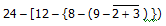We will first remove the bar bracket then circular bracket, then curly bracket and at the last stage we remove the square bracket.

So the expression given above is

= 24 – [12 – {8 – (9 – 2 – 3)}]

= 24 – [12 – {8 – 4}]

= 24 – [12 – 4]

= 24 – 8

= 16

4) The rule of VBODMAS gives us the arrangement according to which an expression is to be simplified. VBODMAS stands for vinculum, brackets, of, division, multiplication, addition and subtraction respectively.

5) Algebraic Identities: The algebraic identities noted below are used as expression formulae:

GENERAL RULES FOR SOLVING PROBLEMS IN ARITHMATIC

1. (a+b) (a-b) = a2 - b2
2. (a+b)2 = a2 + b2 + 2ab
3. (a – b) 2 = a2 + b2 – 2ab
4. (a + b)3 = a3 + b3 + 3ab (a+b)
5. (a – b)3 = a3 – b3 – 3ab (a–b)
6. a2 + b2  =  (a + b)2   – 2ab
7. a3 + b3 = (a+b) (a2 + b2 – ab) = (a+b)3 – 3ab (a+b)
8. a3 - b3  =  (a – b) ( a2 + b2 + ab) =  (a–b)+ 3ab (a-b)
9. a3 + b3 + c3 = (a + b + c) (a2 + b2 + c2 – ab – bc – ca) + 3abc If a + b + c = 0, then a3 + b3 + c3 = 3abc
10. (a+b+c)2 = a2+b2+c2+2ab+2bc+2ca
11. (a+b+c)3 = a3+b3+c3+3a2 (b+c) + 3b2(a+b)+3c2(a+b)+6abc [remember there are ten terms]
12. To find the sum of a and b, given their difference and product:
a + b =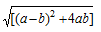13. To find the difference of a and b, given their sum and product:

a – b =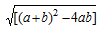QUESTIONS ON SIMPLIFICATION

Generally the types of questions which can be asked for simplification are of the following types.

Type 1

Questions involving expressions in which the items / numbers are either to be added or subtracted. The numbers may be integers or decimals.

Type – II

The numbers to be added or subtracted are fractions. The fractions may be pure or mixed fractions.

Type – III

The numbers or items in the expression are connected with the signs of multiplication or division e.g.

i) 1.6 x 20 x 12 + 30 x 2

ii) 2.21 ÷ 0.7 = ? + 5.5

iii) 4545 ÷ 50 ÷ 5 = ?

Type – IV

These questions contain signs of multiplication, division, percentage, of, etc and also fraction e.g.

i) 35% of 495 + ? = 250

ii)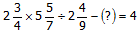iii) 30% 150 + ? of 300 = 40 % of 450

Type V

Square – roots and cube roots along with fractions etc. are there in this type of questions on simplification like.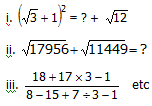Type VI

This type of questions may relate to surds and / or indices like

i) (19.7)5 ÷ (19.7)4 =?

ii) 2310.5 x 235.1 ÷ 231.2 = 23?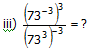Type VII may relate to inequalities or equalities with signs of (<, =, >)

Offer running on EduRev: Apply code STAYHOME200 to get INR 200 off on our premium plan EduRev Infinity!

## Quantitative Techniques for CLAT

61 videos|22 docs|98 tests

,

,

,

,

,

,

,

,

,

,

,

,

,

,

,

,

,

,

,

,

,

;